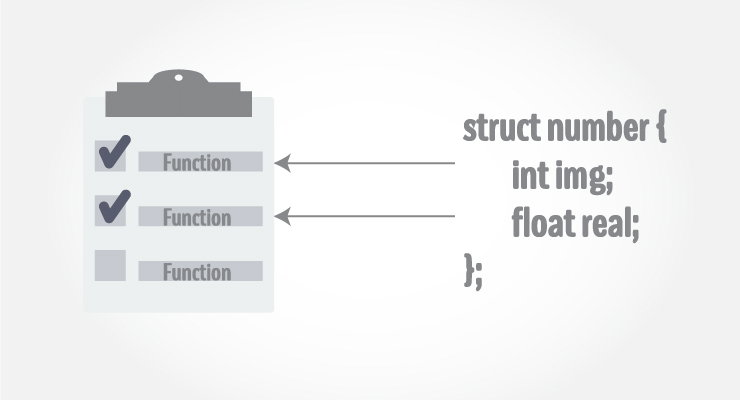# C++ Structure and Function

In this article, you'll find relevant examples to pass structures as an argument to a function, and use them in your program.Structure variables can be passed to a function and returned in a similar way as normal arguments.

## Passing structure to function in C++

A structure variable can be passed to a function in similar way as normal argument. Consider this example:

### Example 1: C++ Structure and Function

``````#include <iostream>
using namespace std;

struct Person
{
char name;
int age;
float salary;
};

void displayData(Person);   // Function declaration

int main()
{
Person p;

cout << "Enter Full name: ";
cin.get(p.name, 50);
cout << "Enter age: ";
cin >> p.age;
cout << "Enter salary: ";
cin >> p.salary;

// Function call with structure variable as an argument
displayData(p);

return 0;
}

void displayData(Person p)
{
cout << "\nDisplaying Information." << endl;
cout << "Name: " << p.name << endl;
cout <<"Age: " << p.age << endl;
cout << "Salary: " << p.salary;
}``````

Output

```Enter Full name: Bill Jobs
Enter age: 55
Enter salary: 34233.4

Displaying Information.
Name: Bill Jobs
Age: 55
Salary: 34233.4```

In this program, user is asked to enter the name, age and salary of a Person inside `main()` function.

Then, the structure variable p is to passed to a function using.

`displayData(p);`

The return type of `displayData()` is `void` and a single argument of type structure Person is passed.

Then the members of structure `p` is displayed from this function.

### Example 2: Returning structure from function in C++

``````#include <iostream>
using namespace std;

struct Person {
char name;
int age;
float salary;
};

Person getData(Person);
void displayData(Person);

int main()
{

Person p;

p = getData(p);
displayData(p);

return 0;
}

Person getData(Person p) {

cout << "Enter Full name: ";
cin.get(p.name, 50);

cout << "Enter age: ";
cin >> p.age;

cout << "Enter salary: ";
cin >> p.salary;

return p;
}

void displayData(Person p)
{
cout << "\nDisplaying Information." << endl;
cout << "Name: " << p.name << endl;
cout <<"Age: " << p.age << endl;
cout << "Salary: " << p.salary;
}
``````

The output of this program is same as program above.

In this program, the structure variable p of type structure Person is defined under `main()` function.

The structure variable p is passed to `getData()` function which takes input from user which is then returned to main function.

`p = getData(p); `

Note: The value of all members of a structure variable can be assigned to another structure using assignment operator = if both structure variables are of same type. You don't need to manually assign each members.

Then the structure variable p is passed to `displayData()` function, which displays the information.